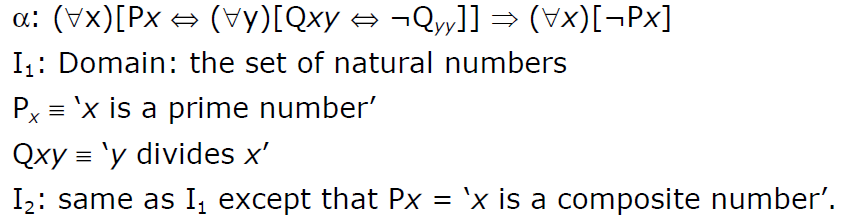# GATE | GATE-CS-2003 | Question 33

Consider the following formula a and its two interpretations I1 and I2Which of the following statements is true?
(A) I1 satisfies α, I2 does not
(B) I2 satisfies α, I1 does not
(C) Neither I2 nor I2 satisfies α
(D) Both I1 and I2 satisfy α

Explanation: First of all, note that, in α, ¬Qyy is always false, because every number divides itself. Also not that rightmost formula (∀x)[¬Px] is always false, because clearly it is not the case that every number is not the prime number (in case of I1), nor it is the case that every number is not the composite number (in case of I2). Also note that, variable x in this expression is not same as variable x in leftside expression, they are independent. In fact, we can rewrite α as α:(∀x)[Px⇔(∀y)[Qxy⇔¬Qyy]]⇒(∀z)[¬Pz].
Let us consider I1 first. So let us assign some value to x, and see if it satisfies α. We can partition assignments of x into 3 parts : when x is prime, when x is composite, when x is 1.

• When x is prime : Px is true, also Qxy is false for all y except 1, because only 1 divides x. So formula Qxy⇔¬Qyy is true for all y except 1, but due to ∀y outside this, whole formula ∀y[Qxy⇔¬Qyy] becomes false, because it would have been true if Qxy⇔¬Qyy was true for every y.
So now [Px⇔(∀y)[Qxy⇔¬Qyy]] becomes false for all x whenever x is prime.
Since for some x (where x is prime), [Px⇔(∀y)[Qxy⇔¬Qyy]] is false, so (∀x)[Px⇔(∀y)[Qxy⇔¬Qyy]] is definitely false, since false⇒ false is true, so α is true in I1, and we don’t need other cases of x.
• Now consider I2. Here also we can argue in the same way as we did in cases of I1, here case of x being composite leads to false⇒false, and so α is also true in I2, hence option (D) is correct.
My Personal Notes arrow_drop_up
Article Tags :

Be the First to upvote.

Please write to us at contribute@geeksforgeeks.org to report any issue with the above content.